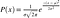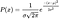# VS Code Math to Image: Write LaTeX Math Equations in GitHub Markdown the Easy Way!

As machine learning engineers or mathematicians, being able to use LaTeX to write math equations is a must for publishing our work on the web. However, rendering math inside Markdown require third party libraries (for instance: MathJax and KaTeX), and not all platforms support LaTeX math rendering. GitHub is one of them. (Medium is another…) 👎

If we want to write math equations inside GitHub README or other Markdown files, we’ll have to find a workaround. Fortunately, GitHub allows adding SVGs (and HTML) directly into our README, which means that we can render our math equations beforehand, and embed SVGs directly inside Markdown files.

So, how can we implement and automate this conversion process? Since most of us use VS Code and/or other editors to publish content to GitHub, me and my friends made a VS Code extension called Math to Image to help you render your math equations like a breeze!

# Installation

Installing Math to Image is quite simple. Just search for vscode-math-to-image or install directly from VS Marketplace.

# Using the extension

After installing the extension, you’ll be able to do a direct, in-place math to image conversion simply by selecting on the equation and select “Math » Image: xxx” in the context menu.

To put you into perspective, for the following equation written as you would normally do in a Markdown file (math equations WILL be rendered in VS Code’s Markdown preview window by default):

$$\ell = \sum_{i}^{N}(y_i - \hat{y}_i)^2 - ||w||_2^2$$

Simply select the 3 lines of code, right click, select either “Math » Image: Insert rendered equation (local)” or “Math » Image: Insert rendered equation (remote)” from the context menu, and we’re done!

“So what’s with the two options: ‘remote’ and ‘local’?”, you may ask. Let me explain.

## Rendering to remote SVGs

Although GitHub won’t render math equations in Markdown files, they actually DO render LaTeX inside Jupyter notebooks. How? Well, GitHub has their own LaTeX rendering server, which takes a encoded math equation, and returns the rendered SVG. That’s exactly what we need!

So, for this extension, we basically took advantage of this feature: utilizing GitHub’s equation rendering server to embed SVG equations in GitHub. (See here for details: A hack for showing LaTeX formulas in GitHub markdown.)

Basically, we can convert a standard LaTeX math equation like the Gaussian Normal Distribution

$$P(x) = \frac{1}{\sigma\sqrt{2\pi}} e^{\frac{-(x-\mu)^2}{2\sigma^2}}$$

… to a rendered image tag with the help of GitHub’s math rendering server, which we can then embed inside GitHub Markdown files directly:

<div align="center"><img src="https://render.githubusercontent.com/render/math?math=P(x)%20%3D%20%5Cfrac%7B1%7D%7B%5Csigma%5Csqrt%7B2%5Cpi%7D%7D%20e%5E%7B%5Cfrac%7B-(x-%5Cmu)%5E2%7D%7B2%5Csigma%5E2%7D%7D%0D"></div>Medium doesn’t allow SVG images, so this is a PNG I converted manually from the rendered SVG equation…XD

## Rendering to local SVGs

Not everywhere accept external SVGs. To circumvent this type of scenario, we can render math equations directly to local SVGs (with MathJax, in our case), and embed these local SVGs into our Markdown as a workaround.

We can convert the same LaTeX math equation…

$$P(x) = \frac{1}{\sigma\sqrt{2\pi}} e^{\frac{-(x-\mu)^2}{2\sigma^2}}$$

… to a local SVG like: svg/e40qQ5G9jw.svg, which will be saved to a dedicated folder called svg, and sourced inside the Markdown file that requires math-embedding.You can barely notice the difference from the equation rendered by GitHub

Both methods render high quality SVGs for you to embed directly inside Markdown. You can choose either way to render your equations.

Interested? Take a look at some examples for more math rendering scenarios, i.e, inline math, aligned environments…That’s all for this article, thanks for reading, and don’t forget to star us at GitHub or leave a five 🌟 rating at VS Marketplace! 💁‍♂️

## More from SpencerWeekly

Nope, not this week.